A system is a set of interrelating, interconnected parts or elements that, together, generate some distinct outcome or behavior over time. In dynamical systems modeling, the behavior that the system exhibits over time is called it’s dynamic.

Stocks and flows are the basic building blocks of system dynamics models. Jay Forrester originally referred to them as “levels” (for stocks) and “rates” (for flows). A stock variable is measured at one specific time and represents a quantity existing at that point in time (say, December 31, 2004), which may have accumulated in the past. A flow variable is measured over an interval of time. Therefore a flow would be measured per unit of time (say a year).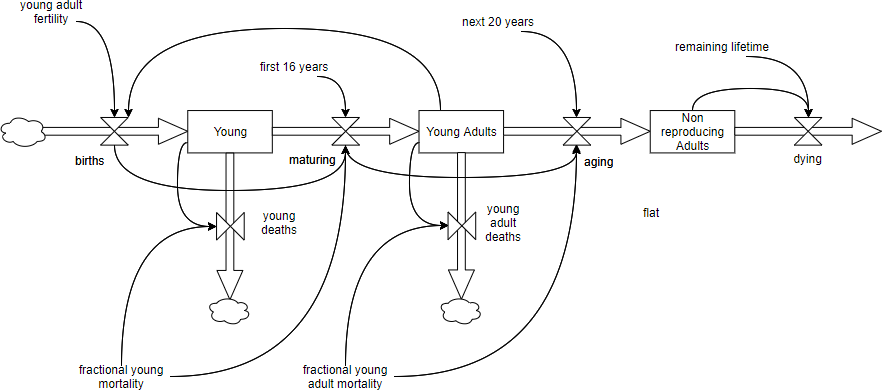Edit this Stock and Flow diagram

## Elements of Stock Flow Diagram

 Name Symbol Description Stock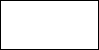·         A stock is accumulated over time by inflows and/or depleted by outflows. Stocks can only be changed via flows. ·         Mathematically a stock can be seen as an accumulation or integration of flows over time – with outflows subtracting from the stock. ·         Stocks typically have a certain value at each moment of time. ·         Example: the number of population at a certain moment. Flow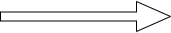·         A flow changes a stock over time. Usually, we can clearly distinguish inflows (adding to the stock) and outflows (subtracting from the stock). ·         Flows typically are measured over a certain interval of time ·         Example: the number of births over a day or month. Flow Rate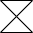·         It is always expressed per some unit time: If these flow into/out of a stock that keeps track of things of type X ·         e.g. Incidence Rates is calculated by accumulating people over a year, revenue is \$/Time, water flow is liters/minute Cloud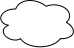Cloud represents either: ·         Source of the flow – when the flow is originated from outside the model ·         Sink of the flow – when the flow sinks Both sources and sinks are assumed to have infinite capacity and do not impose any limitations on the flows. Clouds are drawn as part of the flow element, in case the flow does not flows in/out of some stock. Link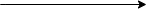·         Link is used to define a dependency between elements of a stock and flow diagram ·         If some element A is mentioned in the equation or initial value of element B, you should first connect these elements with a link going from A to B and only then type the expression in the properties of B.

## Stock and Flow Diagram Example – Rabbits and Foxes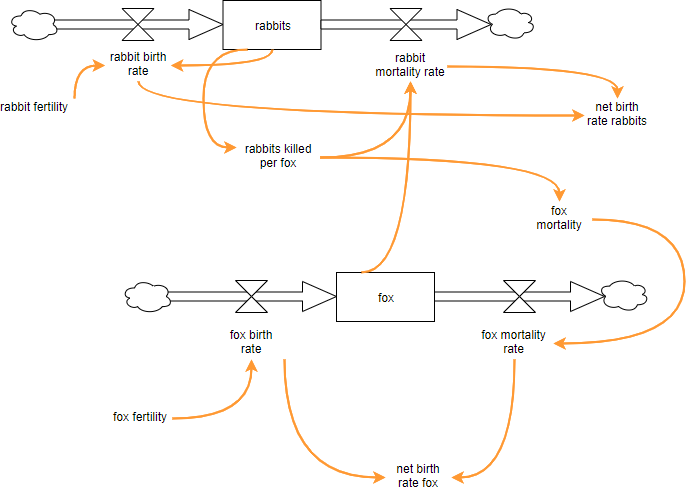Edit this Stock and Flow diagram